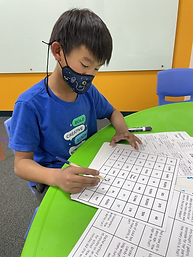Ms. Caitlyn

Target 1​

Lesson Type:

New

Number Operation

:

Fractional Numbers

1:

Use currency symbols (\$ and ₵) appropriately.

2:

Add and subtract currency with decimal points.

5th

Vocabulary:

Currency, Budget

Activities:

• Students stuck to a budget of \$30.00 and bought fruit of various prices for a fruit salad.
• Students calculated the price of all the fruit that they bought.
• Students drew and colored a picture of their fruit salad.Home Exploration

Guiding Questions:Absent Students:

Luca

Target 2

:

1:

Use the standard algorithm for solving multi-digit division equations.

2:

Understand that there can be a remainder in a division problem.

5th

Vocabulary:

Standard Algorithm, Remainder

Activities:

• Students answered division problems to solve a Math Mystery puzzle.Home Exploration

Guiding Questions:Target 3

:

1:

Understand equivalent measurements.

2:

Understand how to convert from one unit to another.

Vocabulary:

U.S. Customary System, Metric System, Conversion

Activities:

• Students reviewed the units of measurement in the U.S. Customary System and the Metric System.
• Students used a unit conversion chart to convert measurements from the U.S. Customary System to the Metric System.
• Students played Slides and Ladders, using measurement conversions to be able to move across the game board.Home Exploration